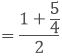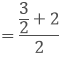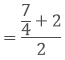×#### Thank you for registering.

One of our academic counsellors will contact you within 1 working day.

Click to Chat

1800-1023-196

+91-120-4616500

CART 0

• 0

MY CART (5)

Use Coupon: CART20 and get 20% off on all online Study Material

ITEM
DETAILS
MRP
DISCOUNT
FINAL PRICE
Total Price: Rs.

There are no items in this cart.
Continue Shopping• Complete JEE Main/Advanced Course and Test Series
• OFFERED PRICE: Rs. 15,900
• View Details

```Chapter 1: Number System Exercise – 1.1

Question: 1

Is 0 a rational number? Can you write it in the form P/Q, where P and Q are integers and Q ≠ 0?

Solution:

Yes, 0 is a rational number and it can be written in P ÷ Q form provided that Q?

0 is an integer and it can be written various forms, for example

0 ÷ 2, 0 ÷ 100, 0 ÷ 95 etc.

Question: 2

Find five rational numbers between 1 and 2

Solution:

Given that to find out 5 rational numbers between 1 and 2

Rational number lying between 1 and 2= 3/2

= 1 < 3/2 < 2

Rational number lying between 1 and 3/2= 5/4

= 1 < 5/4 < 3/2

Rational number lying between 1 and 5/4Rational number lying between 3/2 and 2

= 9/8

= 1 < 9/8 < 5/4

Rational number lying between 3/2 and 2= 7/4

= 3/2 < 7/4 < 2

Rational number lying between 7/4 and 2= 15/8

= 7/4 < 15/8 < 2

Therefore, 1 < 9/8 < 5/4 < 3/2 < 7/4 < 15/8 < 2

Question: 3

Find out 6 rational numbers between 3 and 4

Solution:

Given that to find out 6 rational numbers between 3 and 4

We have,

3 × 7/7 = 21/7 and

4 × 6/6 = 28/7

We know 21 < 22 <  23 < 24 < 25 < 26 < 27 < 28

21/7 < 22/7 < 23/7 < 24/7 < 25/7 < 26/7 < 27/7 < 28/7

3 < 22/7 < 23/7 < 24/7 < 25/7 < 26/7 < 27/7 < 4

Therefore, 6 rational numbers between 3 and 4 are

22/7, 23/7, 24/7, 25/7, 26/7, 27/7

Similarly to find 5 rational numbers between 3 and 4, multiply 3 and 4 respectively with 6/6 and in order to find 8 rational numbers between 3 and 4 multiply 3 and 4 respectively with 8/8 and so on.

Question: 4

Find 5 rational numbers between 3/5 and 4/5

Solution:

Given to find out the 5 rational numbers between 3/5 and 4/5

To find 5 rational numbers between 3/5 and 4/5, 3/5 and 4/5 with 6/6

We have,

3/5 × 6/6 = 18/30

4/5 × 6/6 = 24/30

We know 18 < 19 < 20 < 21 < 22 <  23 < 24

18/30 < 19/30 < 20/30 < 21/30 < 22/30 < 23/30 < 24/30

3/5 < 1930 < 20/30 < 21/30 < 22/30 < 23/30 < 4/5

Therefore, 5 rational numbers between 3/5 and 4/5 are 19/30, 20/30, 21/30, 22/30, 23/30

Question: 5

(i) Every whole number is a rational number

(ii) Every integer is a rational number

(iii) Every rational number is an integer

(iv) Every natural number is a whole number

(v) Every integer is a whole number

(vi) Every rational number is a whole number

Solution:

(i)  True. As whole numbers include and they can be represented

For example - 0/10, 1/1, 2/1, 3/1..... And so on.

(ii) True. As we know 1, 2, 3, 4 and so on, are integers and they can be represented in the form of 1/1, 2/1, 3/1, 4/1.

(iii) False. Numbers such as 3/2, 1/2, 3/5, 4/5 are rational numbers but they are not integers.

(iv) True. Whole numbers include all of the natural numbers.

(v) False. As we know whole numbers are a part of integers.

(vi) False. Integers include -1, -2, -3 and so on....which is not whole number
```### Course Features

• 728 Video Lectures
• Revision Notes
• Previous Year Papers
• Mind Map
• Study Planner
• NCERT Solutions
• Discussion Forum
• Test paper with Video Solution